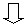# Material: definition (structure)

## Definition

The Material entity is defined by:

• a name
• a type for following physical properties:
• magnetic property B(H) and B(H,T)
• electric property J(E) and J(E,T)
• dielectric property D(E)
• thermal conductivity k(T)
• specific heat ρCP(T)
• specific characteristics belonging to a type

## Name

The name to identify the material is set by the user during the creation of this one.

A comment (optional) can be added to the name.

## Provided types (models)

Various types (models), provided for the physical properties, are presented in chapter Materials: principles. Brief lists of models are presented in the following blocks. For detailed lists, see:

## Outline of the models (1)

An outline of the models for various electromagnetic properties B(H), J(E), D(E) is given in the table below:

B(H) J(E) D(E)
Soft material Hard material
Linear Linear Linear Constant resistivity Linear
Linear complex

Linear complex

Linear with losses

Nonlinear
• Saturation:
• analytic
• analytic + knee
• spline
• Rayleigh

Magnetization:

• analytic
• analytic + knee
• spline
Superconductivity
Hysteresis Jiles-Atherton

## Outline of the models (2)

An outline of the models for various thermal properties k(T), ρCP(T) is given in the table below:

k(T) ρCP(T)
Constant Constant
Linear function of T Linear function of T
Exponential function of T Exponential function of T
Gaussian of T + constant
Gaussian of T + exponential

## Outline of the models (3)

An outline of the models for various electromagnetic properties depending on the temperature B(H,T), J(E,T), D(E,T) is given in the table below:

B(H,T) J(E,T) D(E,T)
Soft material Hard material
Linear Linear No models provided Constant resistivity No models provided
Linear complex - -
Nonlinear

Saturation

• analytic
• analytic + knee
-• Exponential function of T

• Linear function of T
• Exponential function of T